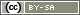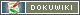This translation is older than the original page and might be outdated. See what has changed.

# registerVariable()

`void registerVariable(string variableName)`

In PHP, a variable is normally only valid within one PHP code element. By using `registerVariable()`, a variable can be made available for all following PHP code elements.

• variableName
A string (in quotation marks) with the name of the variable. This cannot be the variable itself (e.g. `\$item`), a string with the name of the variable (e.g. 'item').

## Example 1

In the following example, on page 1, three items are taken from the items in question “AB01”. Following this, the question is asked with these three items – and, on a later page, question “ABO2” is asked with these exact same three items.

```// Take three items from question AB01
\$itemlist = random_items('AB01', 3);
// Show the respective question
question('AB01', \$itemlist);
// Register variable \$itemlist
registerVariable('itemlist');```

As the variable `\$itemlist` was made available for other pages in the questionnaire, you can just carry on working with `\$itemlist` in another PHP code element.

`question('AB02', \$itemlist);`

## Example 2

On page 4 in the questionnaire, a rather complicated filter is used to determine whether the participant falls into group 1 (prospective clients or clients preparing to do business), or group 2 (current clients). Different questions will be shown later on the in the questionnaire resulting from this classification.

```if (
(value('BB01') == 1) or
((value('BB02') < 3) and (value('BB04') == 2)
) {
\$group = 1;
} else {
\$group = 2;
}
registerVariable('group');```

The variable `\$group` can also be used later on in the questionnaire.

```if (\$group == 1) {
question('BX01');
} else {
question('BX02');
}```
```if (\$group == 1) {
question('BX04', '1-3,5,6');
} else {
question('BX04', '1-5,7,9');
}```

Tip: As the group will also be important in the analysis, the use of `put()` and, on later pages, `value()` would arguably be more effective here.

## Example 3

4 questions (“AB01” to “AB04”) are displayed with their associated text elements (“text1” to “text4) – but in random order. Therefore, an array is shuffled on page 1 of the questionnaire and made available for the following pages with `registerVariable()`. For further details, please see chapter Rotation.

```\$questions = array(
array('AB01', 'text1'),
array('AB02', 'text2'),
array('AB03', 'text3'),
array('AB04', 'text4')
);
shuffle(\$questions);
registerVariable('questions');```

On the following four pages, each question and text element, which can be found in the corresponding position in the array, is displayed.

```text(\$questions);
question(\$questions);```
```text(\$questions);
question(\$questions);```
```text(\$questions);
question(\$questions);```
```text(\$questions);
question(\$questions);```